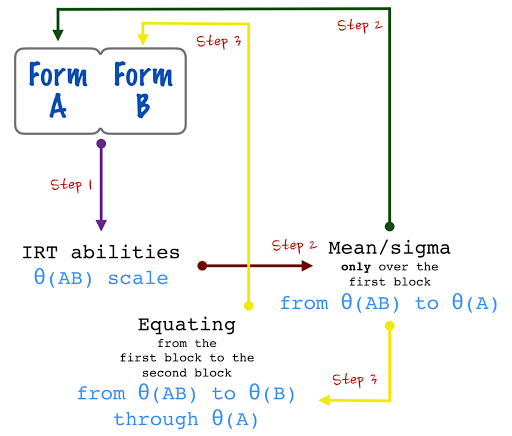Want to share your content on R-bloggers? click here if you have a blog, or here if you don't.

In the assessment of education it is very common to use Item Response Theory in order to produce measures of ability for the students that applied an standardised test. Moreover, if you want to gain comparability between applications you should know that it is not enough to use IRT models but you have to do some work on the design of your test.

First of all, let’s assume you have two test forms (with several common items). The first form (form A) is applied to some students and it is used to define a scale (with specific mean and standard deviation). Then, form A defines the baseline (at this very moment, equating is not considered because there is only a single form). Next, you administer the other form (form B) to different students on a different time. Now, you can define the same mean and sd for the students on that form. However, you cannot find any differences between forms, because of the same mean and sd. In other words, if you just estimate abilities separately the students scores in both forms will not be comparable.Having said that, there is an standardised methodology that will permit to compare results between applications. First of all, you assure that:

• You have a defined baseline.
• You have a design that allows for common items between your forms.
• You keep your modelling techniques the same for all of the remaining applications.
• You equate the scale of those applications in order to detect changes (effects or impacts) in your objective population.

The aim of the equating process is to find comparable differences in ability by using the two forms. In order to achieve this, you gotta equate form B to form A. There is a lot of methodologies that focus on this problem. Here, we show how to perform the calibrated pool method (Lord, 1980) in R. The following chart introduces the necessary steps to achieve this method.

1. Estimate the ability of both groups of students: those who applied form A altogether with those who applied form B.
2. Those abilities found in step 1 are (indeed) in the same joint scale. In this step, you have to use the mean/sigma method to reproduce exactly the same mean and sd of the baseline from the abilities found for the students of the first group.
3. The mean/sigma method in step 3 induced specific constants. Apply those very constants to the second group and find abilities for those students.

Those transformed abilities found in step 4 are comparable with the scale ability defined in the baseline.For the sake of easiness, we will use the dataset LSAT from package ltm and we will adjust a 2PL model for the data. So, we will divide the whole dataset in two blocks: one mimicking the baseline (form A), the other will be used for the equating (form B). The following code is based on step 1.

`rm(list = ls())set.seed(987654) library(ltm)library(mirt)library(dplyr)library(ggplot2) data(LSAT)LSAT <- sample_frac(LSAT) N <- 500 LSAT.0 <- LSAT[1:N,]fit.0 <- mirt(LSAT.0, 1, itemtype = '2PL')z0 <- fscores(fit.0)x0 <- 100 + 10 * scale(z0) mean(x0)sd(x0) coef(fit.0, IRTpars = TRUE, simplify = TRUE)\$items[, c(1, 2)]hist(x0)`

Note that the mean and sd of the baseline is 100 and 10, respectively. We are forcing to the scale of form A to be centred at 100. From now on, we will track changes on abilities regarding this very scale. Let’s assume that we administered the form B (at a later time). Next, we join all of the students (form A along form B) and estimate the common abilities. The following code is based on steps 2 and 3.

`LSAT.01 <- LSAT[1:1000,]fit.01 <- mirt(LSAT.01, 1, itemtype = '2PL')z01 <- fscores(fit.01)z1.0 <- z01[1:N]z1.1 <- z01[(N + 1):1000] # Mean/sigma b1 <- (sd(x0) / sd(z1.0))b0 <- mean(x0) - b1 * mean(z1.0) #Verify that mean and sd are the same on baseline x0.0 <- b0 + b1 * z1.0mean(x0.0) ; mean(x0)sd(x0.0) ; sd(x0) `

Finally, we apply those constants found in step 4 to the joint scale only for the subset of students that applied form B. This scale is absolutely comparable with the baseline scale.

`x1.0 <- b0 + b1 * z1.1mean(x1.0)sd(x1.0)  `
`mean(x1.0) - mean(x0)`

The following plot shows the estimated ability for both applications. Note that both densities are on the same scale although the mean and sd of both forms are not the same.